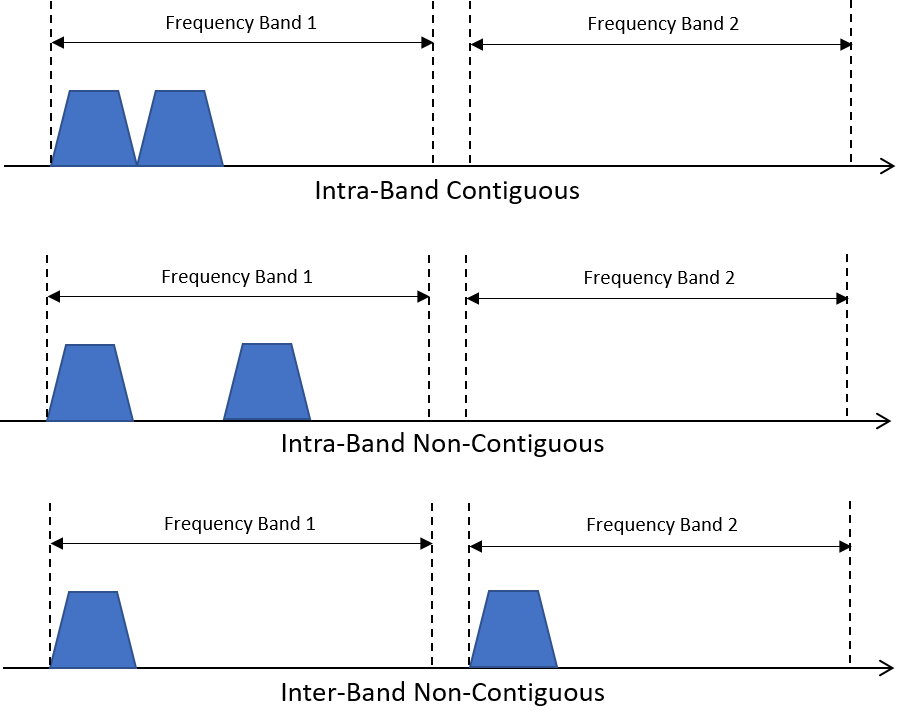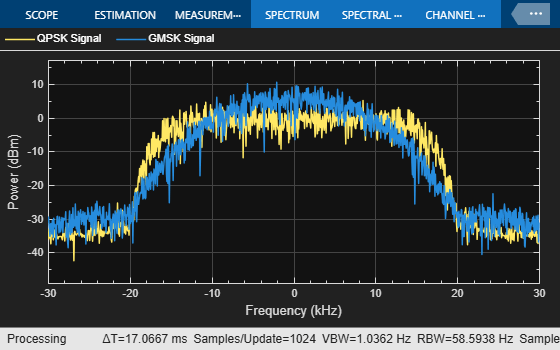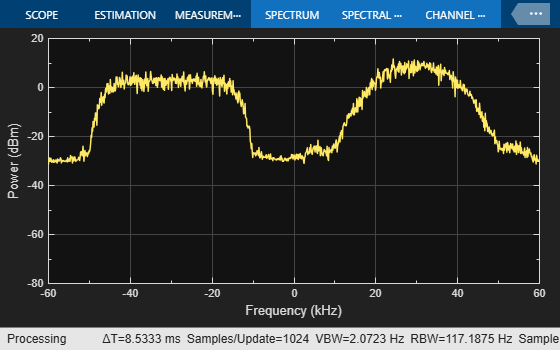# Multiband Signal Generation

This example shows how to generate a multiband signal efficiently using the Communications Toolbox™.

### Introduction

The explosive growth of consumer demand for higher data rates in mobile applications leads to higher transmission rates. Most modern wireless standards include a technique to enhance the data capacity by combining two or more carriers into one data channel. This technique is called carrier aggregation in 5G and LTE terminology, and channel bonding in Wi-Fi® terminology. This figure illustrates three different types of carrier aggregation.### System Setup

This example demonstrates one approach to model carrier aggregation in a baseband simulation. Two baseband signals are generated - one is a QPSK modulated signal and the other is a GMSK modulated signal. Each signal occupies 60 kHz of bandwidth.

A `SignalAggregator` System object™ performs the tasks necessary for carrier aggregation. If the sample rate of the input signals is not high enough, the frequency content will be distorted when the original signals are frequency shifted to produce the desired carrier aggregation. Setting the `InterpolationMode` property to `'Auto'` configures the object to interpolate the two signals and ensure that the resulting signal sample rate is high enough to avoid aliasing. The `info` method of the System object shows the sample rate of the output signal. After the interpolation, the object applies the specified frequency shifts to the signals and combines them into one signal. This block diagram illustrates the `SignalAggregator` processing:### System Simulation

```% Number of simulation iterations nIter = 10; % Modulation order (QPSK modulation) M = 4; % Sample rates Fs1 = 60e3; Fs2 = 240e3; Fs3 = 360e3; qpskTxFilter = comm.RaisedCosineTransmitFilter('RolloffFactor', 0.3, ... 'OutputSamplesPerSymbol', 2); gmskMod = comm.GMSKModulator('BitInput', true', 'SamplesPerSymbol', 2); % Frequency offset for intra-band contiguous aggregation sigCombinerCB = SignalAggregator('InterpolationMode', 'Auto', ... 'FrequencyOffset', [30e3, 90e3], 'SampleRate', Fs1); % Frequency offset for intra-band non contiguous aggregation sigCombinerNCB = SignalAggregator('InterpolationMode', 'Auto', ... 'FrequencyOffset', [30e3, 150e3], 'SampleRate', Fs1); % Scale factor for scope position scopeSF = 0.7; spectrumBB = dsp.SpectrumAnalyzer('Name', 'Baseband Signals', ... 'NumInputPorts', 2, ... 'SampleRate', 60e3, 'Method', 'Filter bank', ... 'AveragingMethod', 'Exponential', 'ShowLegend', true, ... 'ChannelNames', {'QPSK Signal', 'GMSK Signal'}); spectrumBB.Position = scopeSF * spectrumBB.Position; spectrumBB.Position(1) = spectrumBB.Position(1) - ... spectrumBB.Position(3); spectrumCB = dsp.SpectrumAnalyzer('Name', 'Intra-Band Contiguous', ... 'NumInputPorts', 1, ... 'SampleRate', Fs2, 'Method', 'Filter bank', 'AveragingMethod', 'Exponential'); spectrumCB.Position = scopeSF * spectrumCB.Position; spectrumNCB = dsp.SpectrumAnalyzer('Name', 'Intra-Band Non-Contiguous', ... 'NumInputPorts', 1, ... 'SampleRate', Fs3, 'Method', 'Filter bank', 'AveragingMethod', 'Exponential'); spectrumNCB.Position = scopeSF * spectrumNCB.Position; spectrumNCB.Position(1) = spectrumNCB.Position(1) + ... spectrumNCB.Position(3); for k=1:nIter % Generate QPSK signal data = randi([0, M-1], 200, 1); modSig = pskmod(data, M, pi/4, 'gray'); qpskSignal = qpskTxFilter(modSig); % Generate GMSK signal data = randi([0, 1], 200, 1); gmskSignal = gmskMod(data); % Visualize the two signals spectrumBB(qpskSignal, gmskSignal); % Upsample, frequency shift and combine the two signals to model % intra-band contiguous carrier aggregation combinedSignal = sigCombinerCB([qpskSignal, gmskSignal]); % Visualize the resulting signal spectrumCB(combinedSignal); % Upsample, frequency shift and combine the two signals to model % intra-band non contiguous or inter-band non contiguous carrier % aggregation combinedSignal = sigCombinerNCB([qpskSignal, gmskSignal]); % Visualize the resulting signal spectrumNCB(combinedSignal); end release(spectrumBB); release(spectrumCB); release(spectrumNCB); ```### Visualization

Intra-band contiguous aggregation results in a signal that has two original signals, each 60 kHz wide, occupying two contiguous bands of 60 kHz each. In intra-band non-contiguous aggregation, the two signals occupy non-contiguous bands as shown by the gap between the signal spectra in the Intra-Band Non-Contiguous Spectrum Analyzer. Inter-band non-contiguous aggregation can be similarly achieved by appropriate frequency shifts of the signals.

### Summary and Further Exploration

This example illustrates a technique to model the carrier aggregation that is used by most modern wireless communications standards to increase data rates. A System object is used to encapsulate the necessary processing of interpolation, frequency shift and signal combining. You can explore further in various ways:

1. Use baseband signals with different bandwidths. As `SignalAggregator` System object requires all input signals to have the same sample rate, resample one or more signals to bring all baseband signals to the same rate before using `SignalAggregator` System object.

2. Aggregate more than two baseband signals,

3. Use different aggregation bands and carriers to model inter-band non-contiguous aggregation.

Also, explore the `SignalAggregator` System object to study and possibly alter the processing necessary for carrier aggregation. In `'Auto' InterpolationMode`, the `SignalAggregator` System object interpolates the input signals to ensure that the resulting sample rate of the signals is sufficient to not distort the frequency content of the original signals after they are frequency shifted to produce the required carrier aggregation. You can also interpolate the baseband input signals to the rate you desire before using SignalAggregator object and use the `'None' InterpolationMode` of the System object which will not perform any interpolation.

### Appendix

The following System object is used in this example: